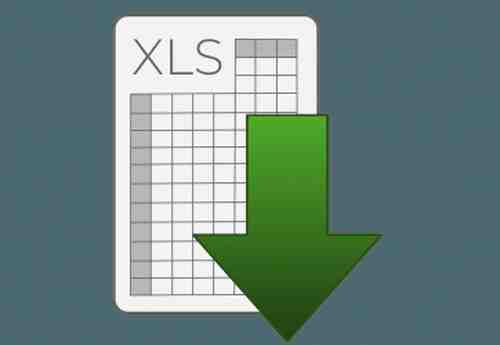# Excel - A macro to perform the automatic numbering of invoicesIn this article we will show you an easy way of doing an automatic numbering in Excel using a MACRO.

How to do it

For example, this MACRO can increment an invoice number. Here, the number is displayed in cell "A1", but it can be changed to your convenience:

Sub Increment_invoice()

Dim num As Integer

Range("A1").Select

num = Range("A1").Value

num = num + 1

Range("A1").Value = num

End Sub

You should also add these lines to the end of a macro so that every time you print your invoice, the next one will be incremented automatically as follows:

Sub PRINT()

ActiveWindow.SelectedSheets.PrintOut Copies:=1, Collate:=True

Dim num As Integer

Range("A1").Select

num = Range("A1").Value

num = num + 1

Range("A1").Value = num

End Sub